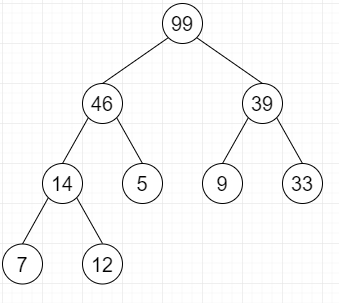# Check if a given Binary Tree is Heap in Python

Suppose we have a binary tree; we have to check whether it is heap or not. The heap has following property: Heap will be a binary tree That tree should be a complete tree (So. all levels except last should be full). Every nodes value of that tree should be greater than or equal to its child node (max-heap).

So, if the input is likethen the output will be true

To solve this, we will follow these steps −

• Define a function number_of_nodes() . This will take root
• if root is null, then
• return 0
• otherwise,
• return(1 + number_of_nodes(root.left) + number_of_nodes(root.right))
• Define a function has_heap_property() . This will take root
• if root.left is null and root.right is null, then
• return True
• if root.right is null, then
• return true when root.val >= root.left.val
• otherwise,
• if (root.val >= root.left.val and root.val >= root.right.val, then
• return(has_heap_property(root.left) and has_heap_property(root.right))
• otherwise,
• return False
• Define a function is_complete_tree() . This will take root,index, node_count
• if root is null, then
• return True
• if index >= node_count, then
• return False
• return(is_complete_tree(root.left, 2 * index + 1, node_count) and is_complete_tree(root.right, 2 * index + 2, node_count))
• From the main method do the following −
• node_count := number_of_nodes()
• if is_complete_tree(root, 0, node_count) and has_heap_property(root) is non-zero, then
• return True
• otherwise,
• return False

## Example

Let us see the following implementation to get better understanding −

Live Demo

class TreeNode:
def __init__(self, value):
self.val = value
self.left = None
self.right = None
def number_of_nodes(self, root):
if root is None:
return 0
else:
return (1 + self.number_of_nodes(root.left) + self.number_of_nodes(root.right))
def has_heap_property(self, root):
if (root.left is None and root.right is None):
return True
if root.right is None:
return root.val >= root.left.val
else:
if (root.val >= root.left.val and
root.val >= root.right.val):
return (self.has_heap_property(root.left) and self.has_heap_property(root.right))
else:
return False
def is_complete_tree(self, root,index, node_count):
if root is None:
return True
if index >= node_count:
return False
return (self.is_complete_tree(root.left, 2 * index + 1, node_count) and self.is_complete_tree(root.right, 2 * index + 2, node_count))
def is_heap(self):
node_count = self.number_of_nodes(self)
if (self.is_complete_tree(self, 0, node_count) and self.has_heap_property(self)):
return True
else:
return False
root = TreeNode(99)
root.left = TreeNode(46)
root.right = TreeNode(39)
root.left.left = TreeNode(14)
root.left.right = TreeNode(5)
root.right.left = TreeNode(9)
root.right.right = TreeNode(33)
root.left.left.left = TreeNode(7)
root.left.left.right = TreeNode(12)
print(root.is_heap())

## Input

root = TreeNode(99)
root.left = TreeNode(46)
root.right = TreeNode(39)
root.left.left = TreeNode(14)
root.left.right = TreeNode(5)
root.right.left = TreeNode(9)
root.right.right = TreeNode(33)
root.left.left.left = TreeNode(7)
root.left.left.right = TreeNode(12)

## Output

True